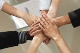# Percentage of total

GridTop achievementsRank 1
Derek asked on 15 Oct 2011, 11:55 PM
Is it possible to show a calculated column which is the percentage of the total for that grid column? let's say I have a data tablie like

[Item],[Quantity]
item A, 3
Item B,5
Item C, 2

I can show a total row, but what I want to do is to show the percentage of total for each row, ie
===
[Item],[Quantity],[%]
Item A, 3, 30%
...
Total, 10
===

Is this possible?

## 3 Answers, 1 is accepted

0Princy
Top achievementsRank 1
answered on 17 Oct 2011, 07:19 AM
Hello Derek,

You can try the following to calculate percentage for each row.

ASPX:
`<``telerik:GridCalculatedColumn` `DataFormatString``=``"{0:d}%"`  `UniqueName``=``"Percentage"` `HeaderText``=``"Percentage"` `Expression``=``"{0}*10"` `DataFields``=``"Quantity"``>`
`</``telerik:GridCalculatedColumn``>`

Thanks,
Princy.
0Derek
Top achievementsRank 1
answered on 18 Oct 2011, 10:51 PM
Thanks for the response, but I don't think I made my question clear enough.

What I'd like to do is to show the percentage that the current row is of the total for the entire table. Let's say I have 10 rows in the table, and the bottom-right total is 1000. If I have a total of 150 in my first row, I'd like to show that the total of the first row is 150/1000, or 15% of the overall total. I don't know if there is a way to access the footer totals from within the individual row.
0
AcceptedPrincy
Top achievementsRank 1
answered on 19 Oct 2011, 07:47 AM
Hello Derek,

You can try the following to find the percentage of total for each row.

C#:
`protected` `void` `RadGrid1_PreRender(``object` `sender, EventArgs e)`
`{`
`  ``GridFooterItem footer1 = (GridFooterItem)RadGrid1.MasterTableView.GetItems(GridItemType.Footer);`
`  ``string` `value1 = footer1[``"quantity"``].Text;`
`  ``double` `footervalue2 = Convert.ToDouble(value1.Split(``':'``));``//to get the value only.`
`  ``foreach` `(GridDataItem item ``in` `RadGrid1.Items)`
`  ``{`
`   ``string` `qitem = item[``"quantity"``].Text;`
`   ``double` `qitem1 = ``double``.Parse(qitem);`
`   ``double` `per = qitem1 / footervalue2;`
`   ``string` `v2 = per + ``"%"``;`
`   ``item[``"Percentage1"``].Text = v2;`
`   ``}`
`}`

Thanks,
Princy.
Tags
GridDerek
Top achievementsRank 1Rank 1Rank 1